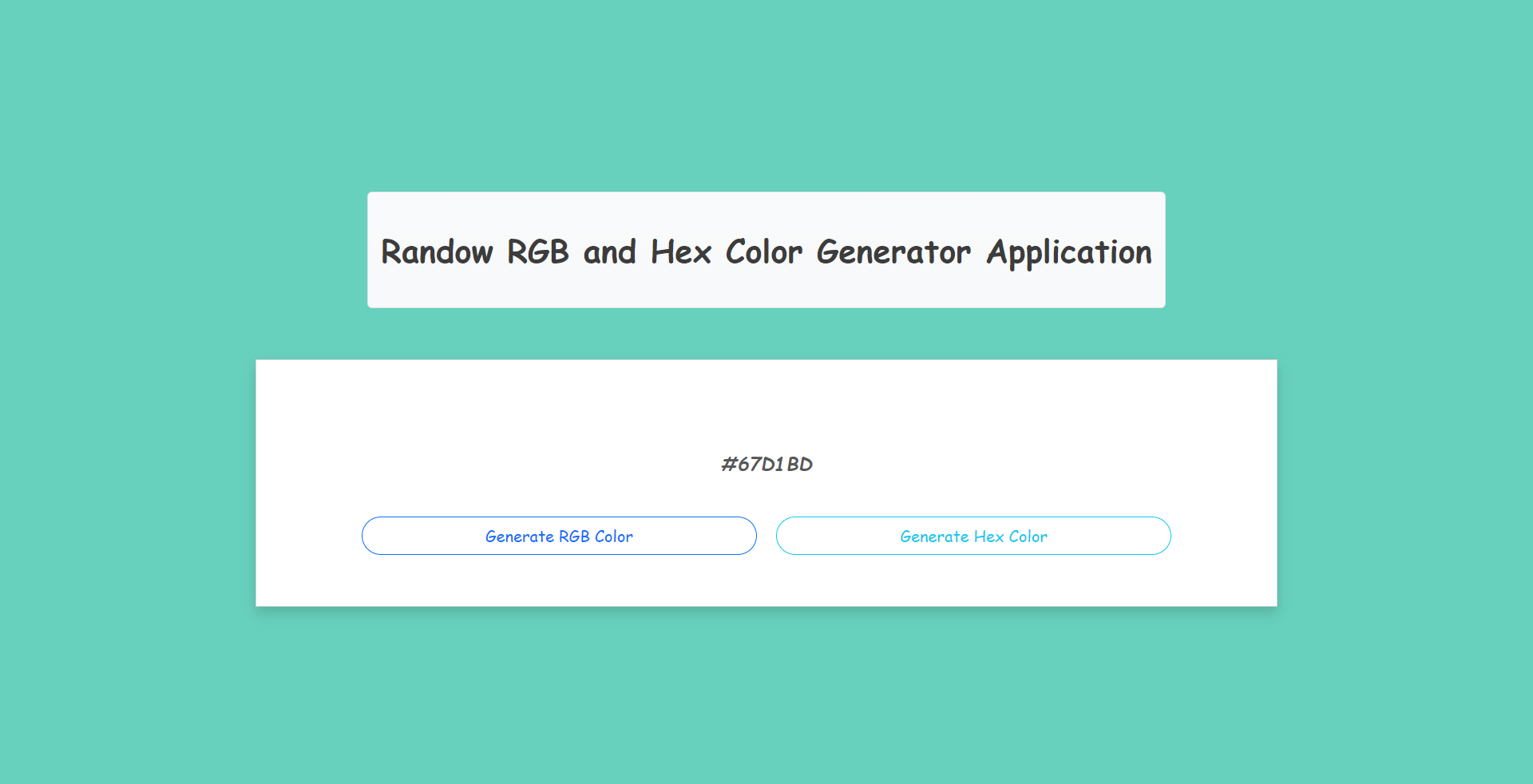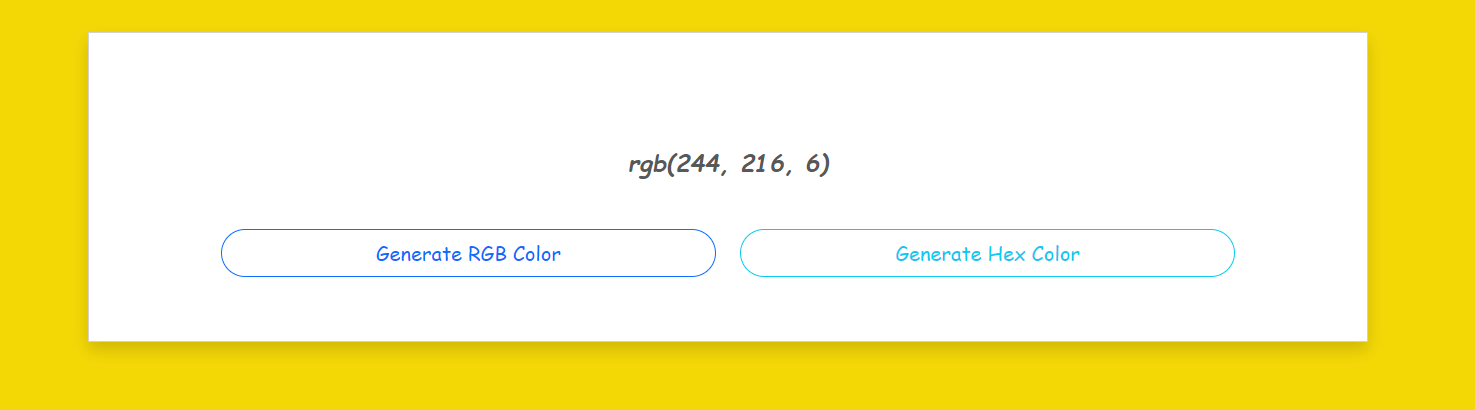# Random RGB and Hex Color Generator Application in JavaScript Free Source Code

## Introduction

This simple project is a Random RGB and Hex Color Generator Application. This is a web-based application project developed in JavaScript, jQuery, HTML, and CSS. The main purpose of this application is to generate a random RGB and Hex Color. This application can help new programmers or students to have a reference on how to generate random values in JavaScript. The application has a simple user interface using the Bootstrap 5 Framework and consists of simple and user-friendly features and functionalities.

## About the Random RGB and Hex Color Generator Application

I developed the Application using the following:

Logic and Method

This Random RGB and Hex Color Generator Application contain only a very simple and small scope of functions. Users can generate either an RGB or Hex color code. On this application, the user can simply generate color code by clicking either the "Generate RGB Color" or the "Generate Hex Color" buttons. Each of these buttons triggers the application to generate a random color and display the color by changing the Page Background Color with the generated color. Then, the application also returns the generated color code above the buttons.

## Script Used for Generating the Color

### RGB Color Code

`	// Red Random Value    var r = Math.ceil(Math.random() * 255);    // Green Random Value    var g = Math.ceil(Math.random() * 255);    // Blue Random Value    var b = Math.ceil(Math.random() * 255);     // Generated Random RGB Color     var rgb = `rgb(\${r}, \${g}, \${b})`;`

### Hex Color Code

`	 // HEX Values    var hex_values = ['0', '1', '2', '3', '4', '5', '6', '7', '8', '9', 'A', 'B', 'C', 'D', 'F'];     //  Hex Generated Values    var v1 = hex_values[Math.ceil(Math.random() * (Object.keys(hex_values).length - 1))]    var v2 = hex_values[Math.ceil(Math.random() * (Object.keys(hex_values).length - 1))]    var v3 = hex_values[Math.ceil(Math.random() * (Object.keys(hex_values).length - 1))]    var v4 = hex_values[Math.ceil(Math.random() * (Object.keys(hex_values).length - 1))]    var v5 = hex_values[Math.ceil(Math.random() * (Object.keys(hex_values).length - 1))]    var v6 = hex_values[Math.ceil(Math.random() * (Object.keys(hex_values).length - 1))]     // Generated Random HEX Color     var hex = `#\${v1}\${v2}\${v3}\${v4}\${v5}\${v6}``

## Features

• Generate RGB Color Code
• Generate Hex Color Code
• Changing Page Background Color
• Returns the Generated Background Color

The source code was developed only for educational purposes only. You can download the source code for free and modify it the way you wanted.

## System Snapshots of some Features

### Page User Interface### Sample Generated RGB Color Code### Sample Generated Hex Color Code## How to Run ??

3. Locate the index.html file.
4. Open the index.html file with your preferred browsers such as Chrome Browser or you can directly browse the index.html file path on your browser. i.e. file:///C:/personal/desktop/js-color_generator/index.html

### DEMO VIDEO

That's it. You can now explore the features and functionalities of this Random RGB and Hex Color Generator Application in JavaScript. I hope this will help you with what you are looking for and you'll find something useful for your future projects.

Explore more on this website for more Free Source Codes and Tutorials.

### Enjoy :)

Note: Due to the size or complexity of this submission, the author has submitted it as a .zip file to shorten your download time. After downloading it, you will need a program like Winzip to decompress it.

Virus note: All files are scanned once-a-day by SourceCodester.com for viruses, but new viruses come out every day, so no prevention program can catch 100% of them.